# INTRODUCTION

Storms and other severe weather events can cause both public health and economic problems for communities and municipalities. Many severe events can result in fatalities, injuries, and property damage, and preventing such outcomes to the extent possible is a key concern.

This project involves exploring the U.S. National Oceanic and Atmospheric Administration’s (NOAA) storm database. This database tracks characteristics of major storms and weather events in the United States, including when and where they occur, as well as estimates of any fatalities, injuries, and property damage.

# SYNOPSIS

The data has been processed to answer the following questions :

1. Across the United States, which types of events are most harmful with respect to population health?

2. Across the United States, which types of events have the greatest economic consequences?

We have used the total and average formula for all the variables to find which has caused the most damage from the events in the database starting in the year 1950 and ending on November 2011.The results have been shown through bar plots and the analysis is given below.

SETTING GLOBAL OPTIONS:

library(dplyr)
library(RColorBrewer)
library(ggplot2)

data<- read.csv("final.bz2", header=TRUE, sep = ",") #file name is final.bz2

names(data) #Checking out the names of the data set
##   "STATE__"    "BGN_DATE"   "BGN_TIME"   "TIME_ZONE"  "COUNTY"
##   "COUNTYNAME" "STATE"      "EVTYPE"     "BGN_RANGE"  "BGN_AZI"
##  "BGN_LOCATI" "END_DATE"   "END_TIME"   "COUNTY_END" "COUNTYENDN"
##  "END_RANGE"  "END_AZI"    "END_LOCATI" "LENGTH"     "WIDTH"
##  "F"          "MAG"        "FATALITIES" "INJURIES"   "PROPDMG"
##  "PROPDMGEXP" "CROPDMG"    "CROPDMGEXP" "WFO"        "STATEOFFIC"
##  "ZONENAMES"  "LATITUDE"   "LONGITUDE"  "LATITUDE_E" "LONGITUDE_"
##  "REMARKS"    "REFNUM"

# Preparing the data set:

data1<- data[,c(7,8,22,23,24,25,26,27,28)]

Finding the different variable names in the data:

unique(data1$CROPDMGEXP) ##  "" "M" "K" "m" "B" "?" "0" "k" "2" unique(data1$PROPDMGEXP)
##   "K" "M" ""  "B" "m" "+" "0" "5" "6" "?" "4" "2" "3" "h" "7" "H" "-" "1" "8"

MAKING COLORS

pal <- element_text(colour = "red")
gal <- element_text(colour = "blue", hjust = 0.5)
jal <- element_text(colour = "dark green")
sal <- element_text(colour = "orange", hjust = 0.5)
tal <- element_text(colour = "brown")
ral <- element_text(colour = "magenta", hjust = 0.5)

dal <- brewer.pal(8, "Spectral")
hal <- brewer.pal(8, "BuGn")
fal <- brewer.pal(8, "Set2")
nal <- brewer.pal(8, "Accent")

# DATA PROCESSING:

# CHANGING THE VARIABLE NAMES I.E. "M", "B" WITH NUMERIC VALUES

library(plyr)
## ------------------------------------------------------------------------------
## You have loaded plyr after dplyr - this is likely to cause problems.
## If you need functions from both plyr and dplyr, please load plyr first, then dplyr:
## library(plyr); library(dplyr)
## ------------------------------------------------------------------------------
##
## Attaching package: 'plyr'
## The following objects are masked from 'package:dplyr':
##
##     arrange, count, desc, failwith, id, mutate, rename, summarise,
##     summarize
data1$PROPDMGEXP <-mapvalues(data1$PROPDMGEXP,from = c("K", "M", "",  "B", "m", "+", "0",
"5", "6", "?", "4", "2", "3", "h", "7", "H", "-", "1", "8"),
to =  c(10^3, 10^6, 1, 10^9, 10^6, 0,1,10^5,10^6,0, 10^4,10^2,10^3,10^2,10^7,10^2,0,10,10^8))

data1$CROPDMGEXP<- mapvalues(data1$CROPDMGEXP, from = c("", "M","K", "m", "B", "?", "0", "k", "2"),
to = c(1,10^6,10^3,10^6,10^9,0,1,10^3,10^2))

#CHANGING VALUES TO BILLIONS

data1$proptotal<-data1$PROPDMG * as.numeric(as.character(data1$PROPDMGEXP)) data1$proptotal<- data1$proptotal/1000000000 data1$croptotal<- data1$CROPDMG * as.numeric(as.character(data1$CROPDMGEXP))

data1$croptotal<- data1$croptotal/1000000000 

Variables used:

EVTYPE: Event Type (Tornadoes, Flood, ….)

FATALITIES: Number of Fatalities

INJURIES: Number of Injuries

PROGDMG: Property Damage

PROPDMGEXP: Units for Property Damage (magnitudes - K- thousands,B- Billions,M- Millions)

CROPDMG: Crop Damage

CROPDMGEXP: Units for Crop Damage (magnitudes - K,BM,B)

# MOST HARMFUL WITH RESPECT TO HEALTH:

SUM FOR INJURIES

datainj<- data1%>%
group_by(EVTYPE)%>%
summarise(Injured = sum(INJURIES))
## summarise() ungrouping output (override with .groups argument)
datainj<- datainj[order(-datainj$Injured),] datainj<- datainj[1:8,] barplot(datainj$Injured, names.arg = datainj$EVTYPE,las=3, xlab = "", ylab = "Sum Of Injuries", main = "TOTAL INJURIES", space = 0.4, col = fal)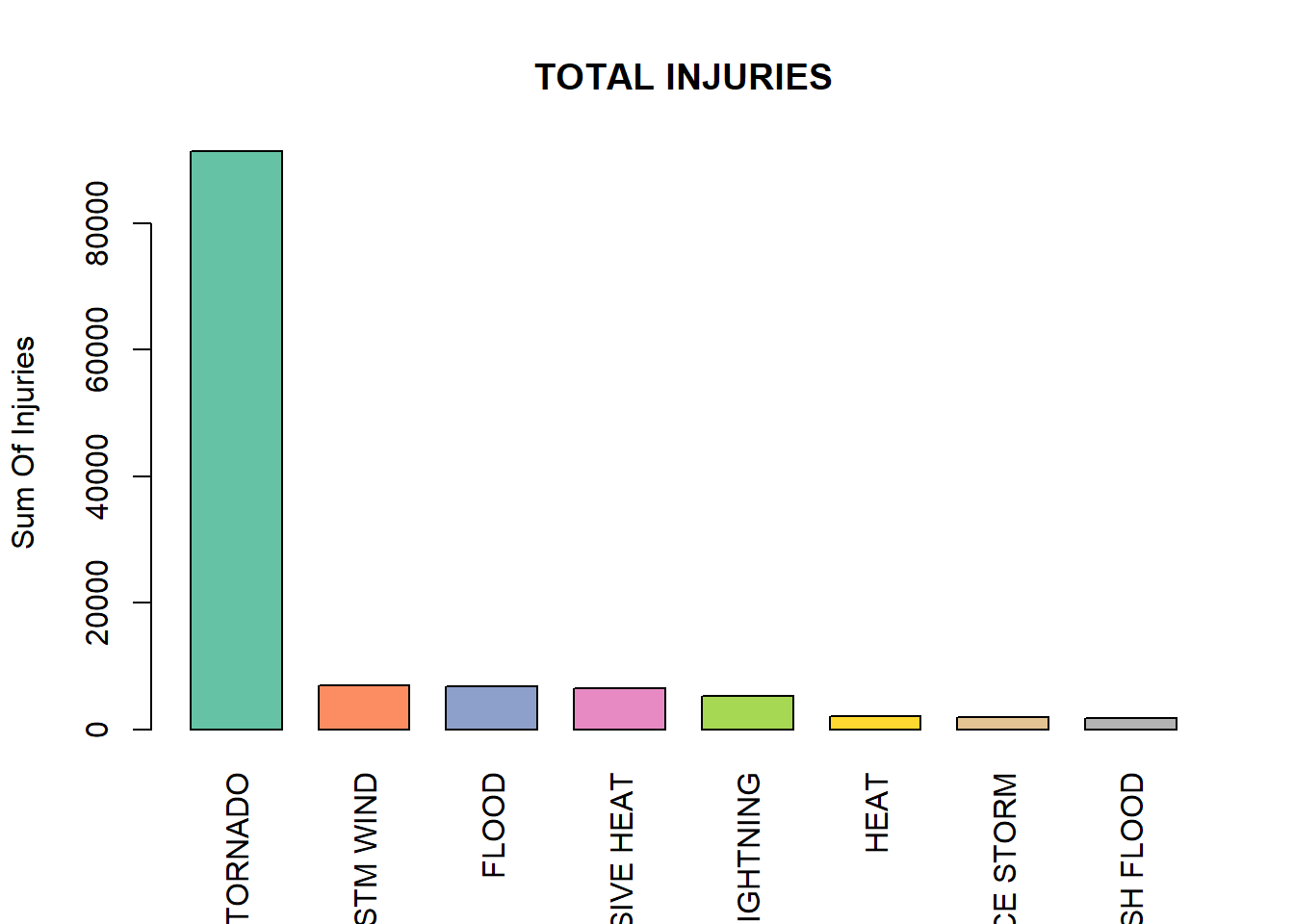MEAN FOR INJURIES datameaninj<- data1%>% group_by(EVTYPE)%>% summarise(MeanInjured = mean(INJURIES)) ## summarise() ungrouping output (override with .groups argument) datameaninj<- datameaninj[order(-datameaninj$MeanInjured),]
datameaninj<- datameaninj[1:8,]

barplot(datameaninj$MeanInjured, names.arg= datameaninj$EVTYPE  ,las=3, xlab = "",
ylab = "Average number Of Injuries", main = "MEAN INJURIES", space = 0.4, col = hal)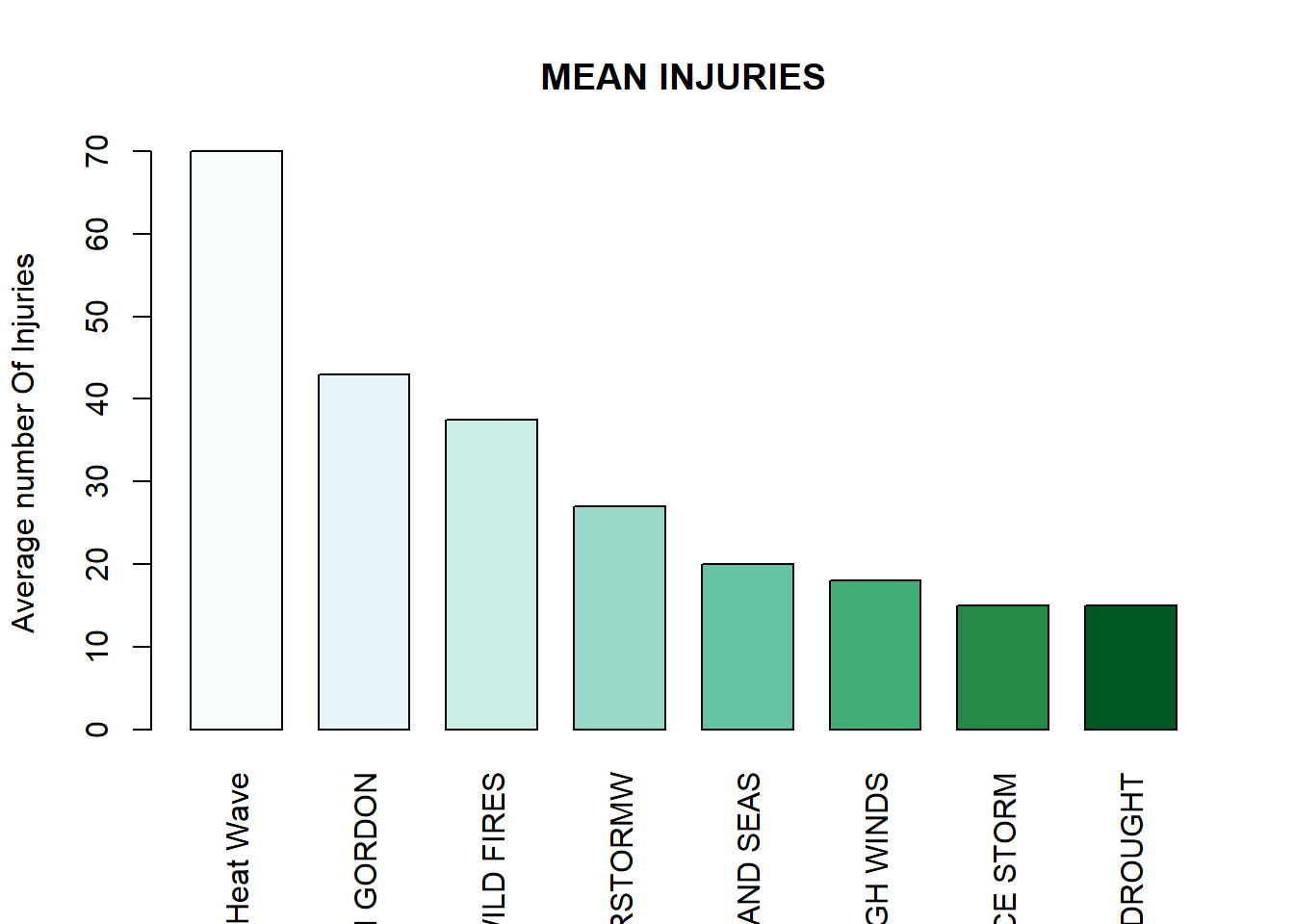SUM FOR FATALITIES

datafat<- data1%>%
group_by(EVTYPE)%>%
summarise(Fatalities = sum(FATALITIES))
## summarise() ungrouping output (override with .groups argument)
datafat<- datafat[order(-datafat$Fatalities),] datafat<- datafat[1:8,] g<- ggplot(datafat, aes(x = reorder(EVTYPE, -Fatalities),y = Fatalities)) g+ geom_bar(stat = "identity", aes(fill=dal))+ theme(axis.text.x = element_text(angle = 90, vjust = 0.5, hjust = 0.5, color = "blue"), axis.text = tal, axis.title = ral, plot.title =gal, legend.position = "none") + labs(title = "TOTAL FATALITIES OVER THE YEARS", x = "EVENT TYPE", y = "Sum of Fatalities")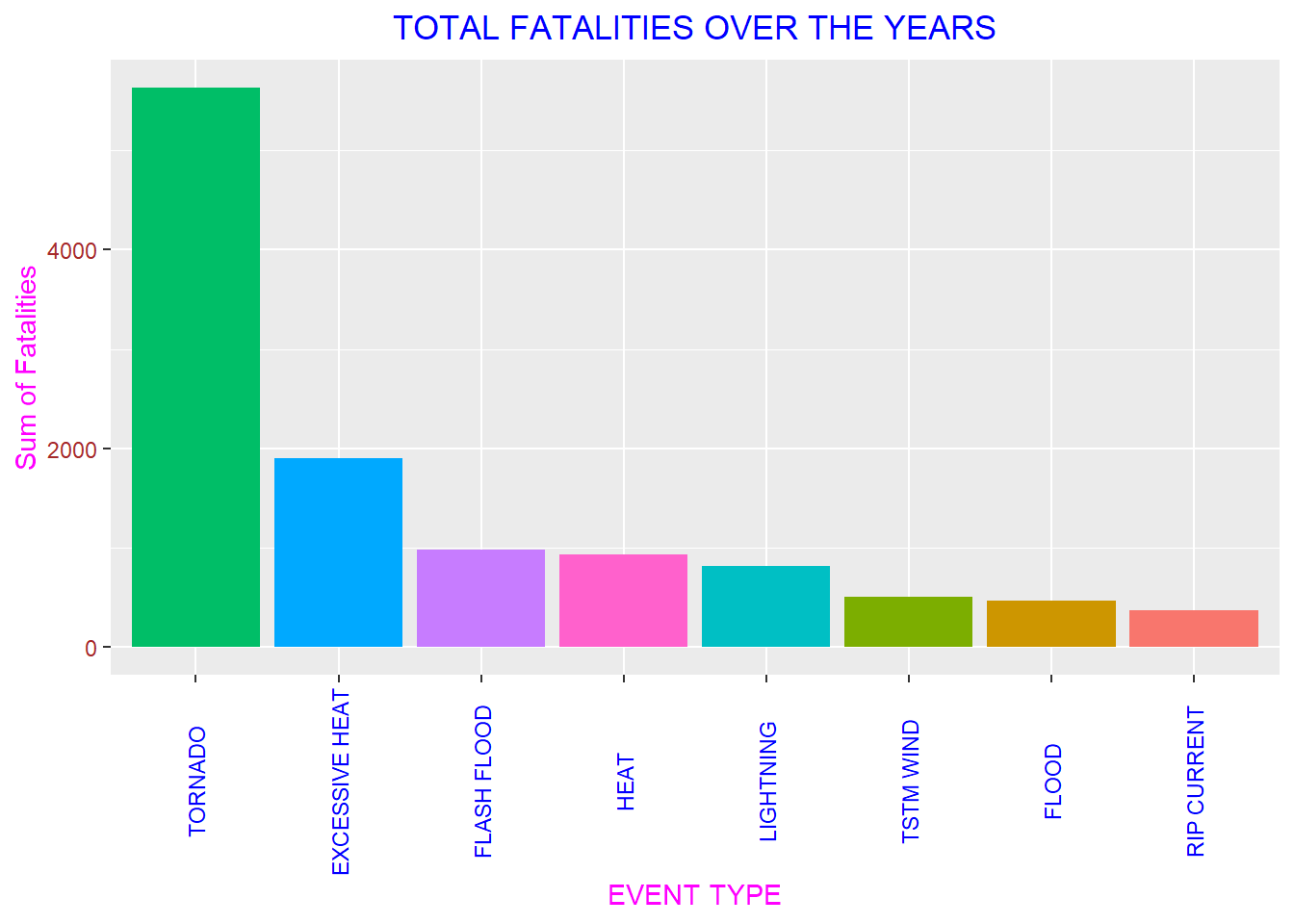MEAN FOR FATALITIES datameanfat<- data1%>% group_by(EVTYPE)%>% summarise(meanFAt= mean(FATALITIES)) ## summarise() ungrouping output (override with .groups argument) datameanfat<- datameanfat[order(-datameanfat$meanFAt),]
datameanfat<- datameanfat[1:8,]

g<- ggplot(datameanfat, aes(x = reorder(EVTYPE, -meanFAt),y = meanFAt))

g+ geom_bar(stat = "identity", aes(fill = fal))+ theme(axis.text.x = element_text(angle = 90, vjust = 0.5, hjust = 0.5, colour = "dark green" ), axis.text.y = pal,axis.title = gal, plot.title = ral, legend.position = "none") +
labs(title = "MEAN FATALITIES OVER THE YEARS", x = "EVENT TYPE", y = "Mean of Fatalities")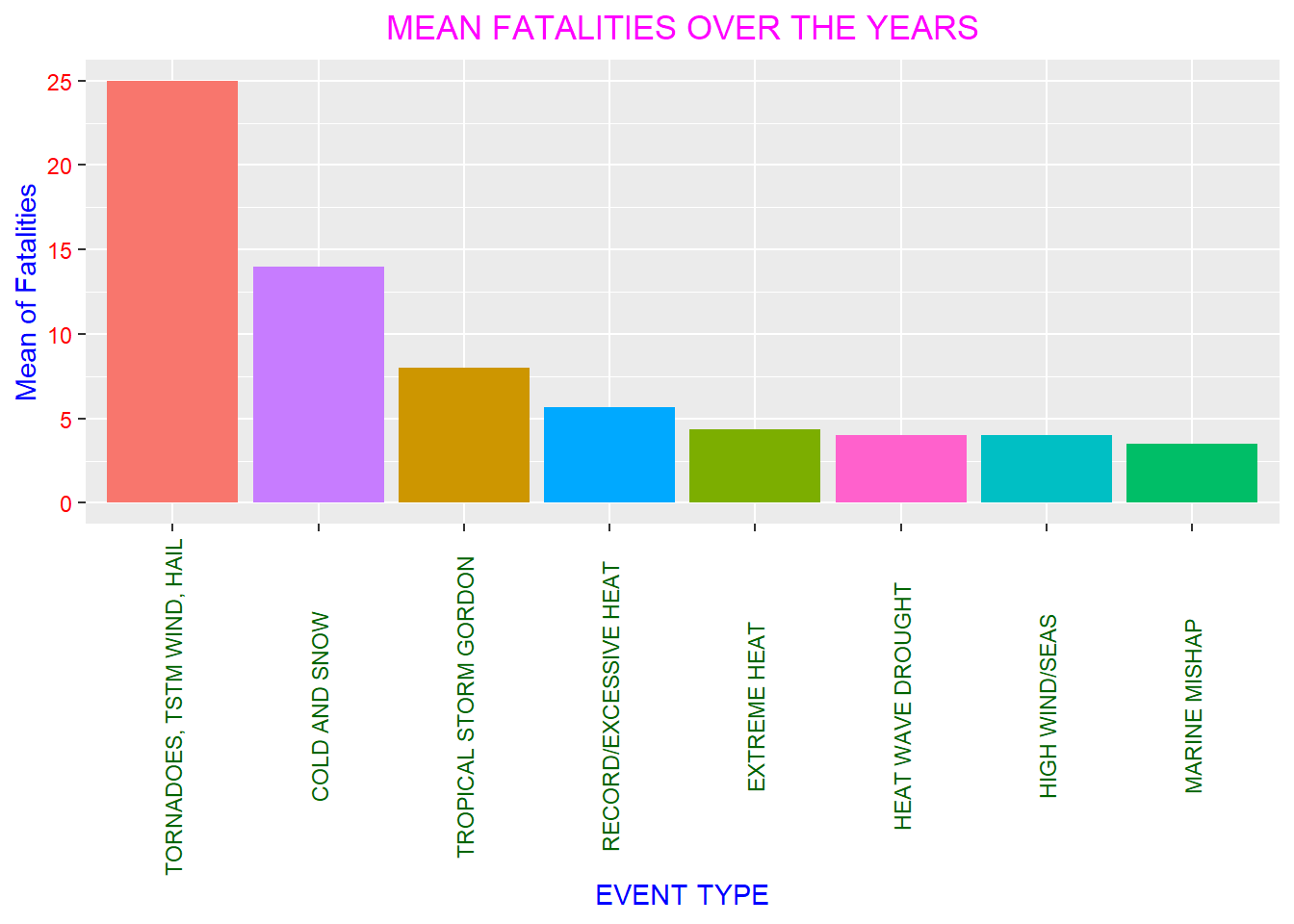# ECONOMIC CONSEQUENCES

Total Crop Damage

datacrop<- data1%>%
group_by(EVTYPE)%>%
summarise(Damage = sum(croptotal))
## summarise() ungrouping output (override with .groups argument)
datacrop<- datacrop[order(-datacrop$Damage),] datacrop<- datacrop[1:8,] g<- ggplot(datacrop, aes(x = reorder(EVTYPE, -Damage),y = Damage )) g+ geom_bar(stat = "identity", aes(fill=nal))+ theme_classic() + theme(axis.text.x = element_text(angle = 90, vjust = 0.5, hjust = 0.5, colour = "maroon" ),axis.text.y = pal, axis.title = jal, plot.title = sal, legend.position = "none") + labs(title = "TOTAL CROP DAMAGE OVER THE YEARS", x = "EVENT TYPE", y = "Sum of Crops damaged (in Billions)")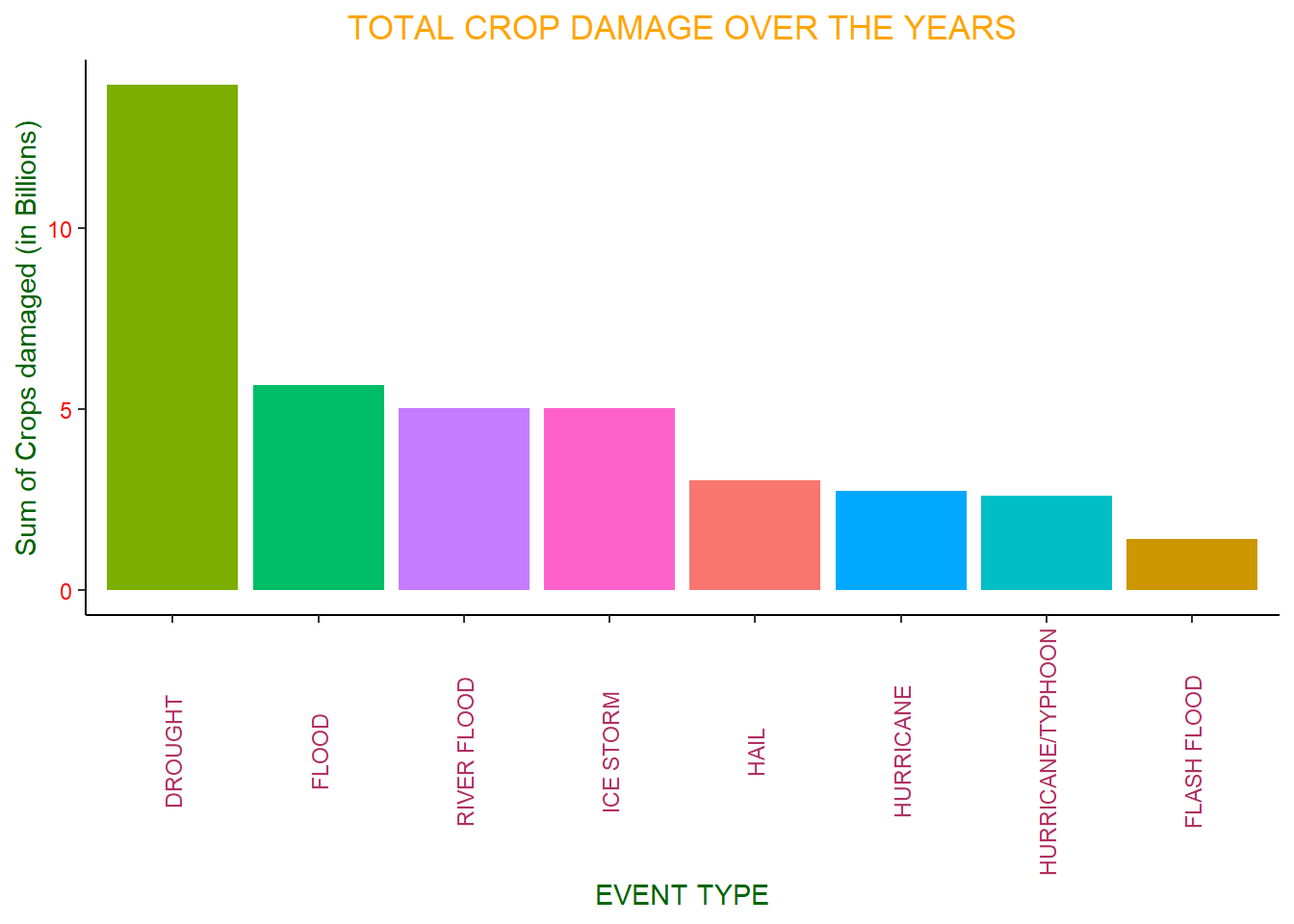Total Property Damage dataprop<- data1%>% group_by(EVTYPE)%>% summarise(Damage = sum(proptotal)) ## summarise() ungrouping output (override with .groups argument) dataprop<- dataprop[order(-dataprop$Damage),]
dataprop<- dataprop[1:8,]

g<- ggplot(dataprop, aes(x = reorder(EVTYPE, -Damage),y = Damage ))

g+ geom_bar(stat = "identity", aes (fill= hal))+ theme_bw()+theme(axis.text.x = element_text(angle = 90, vjust = 0.5, hjust = 0.5, colour = "orange"), axis.text.y = jal, axis.title = gal, plot.title = gal, legend.position = "none") + labs(title = "TOTAL PROPERTY DAMAGE OVER THE YEARS",x = "EVENT TYPE", y= "Total number of property damaged (in Billions)")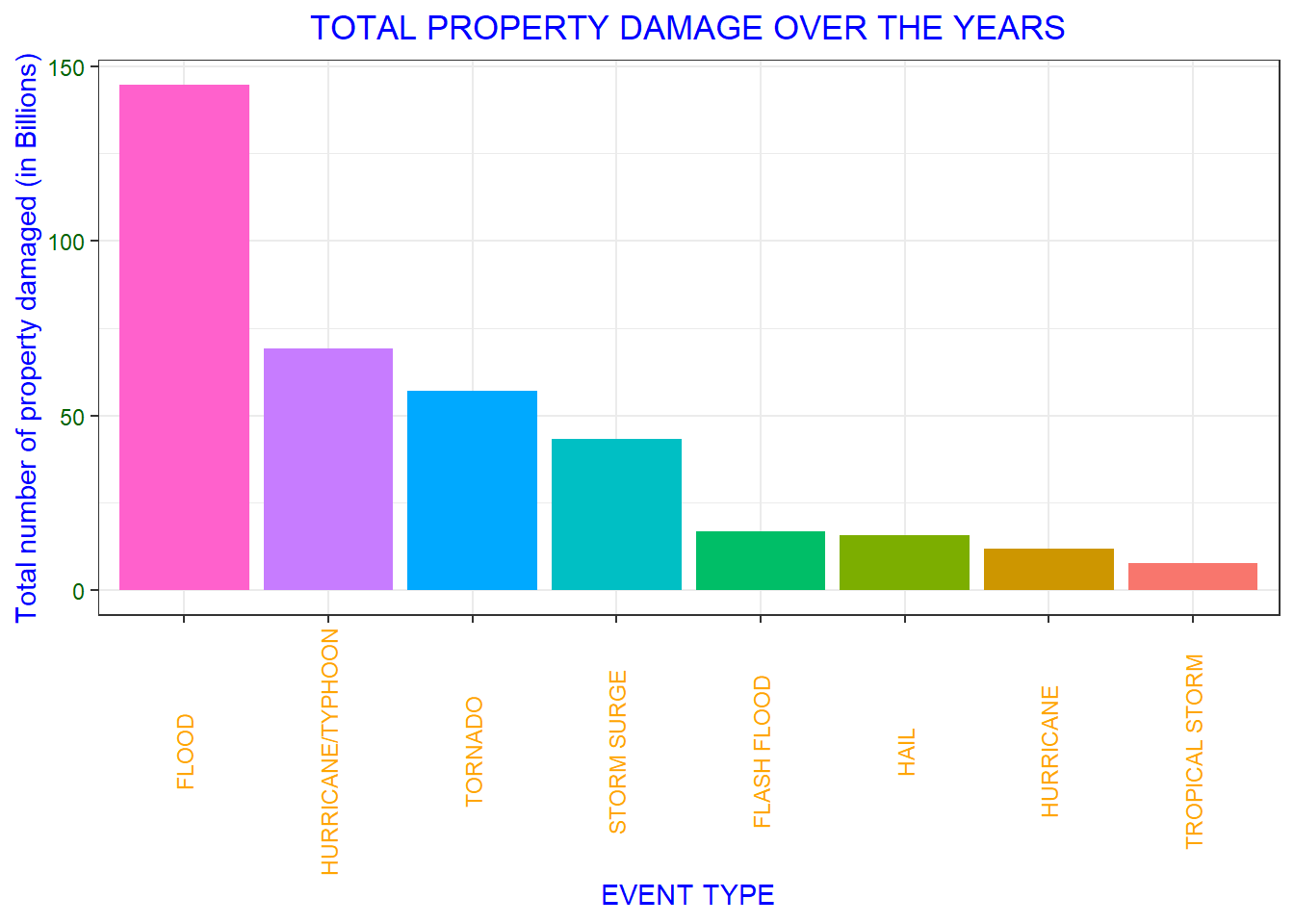# RESULTS:

1. HEALTH PROBLEMS:

Injuries:

The total shows that over 8,000 people have dies due to tornadoes, which is a clear winner for total injuries over the year.

The mean shows that Heat Wave occurs more and injures people whereas tornadoes doesn’t even fall in top 8.

ANALYSIS : Tornadoes injures the most people but they do not occur frequently or sometimes are even dodged without injuries.

Fatalities:

The total shows that over 5,000 fatalities have occurred due to tornadoes,

The mean shows that Tornadoes, TSTM, Wind, Hail have caused approx. 25 fatalities per year.

ANALYSIS : Tornadoes are much more likely to cause higher fatalities.

2. ECONOMIC PROBLEMS:

Crop Damage:

The total shows that Droughts have caused the most crop damage (over 10 Billion Dollars) over the years

Property Damage:

The total shows that Floods have caused over 100 Billion Dollars damage to properties.